•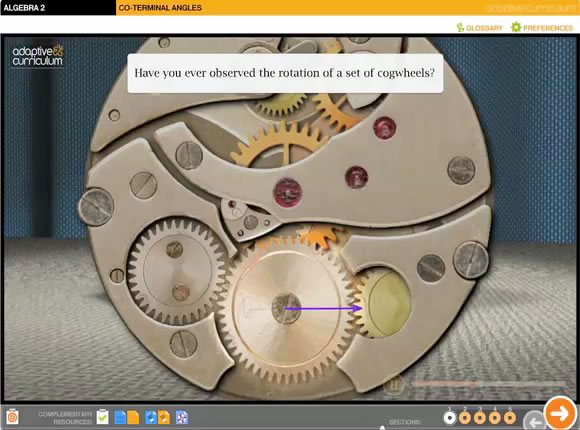Concept Development
Students develop a mathematical concept by placing it in context and exploring its basic principles. Students build knowledge by conducting trials, exploring key examples, and making observations in an interactive and contextually rich environment. Instructive, timely feedback guides students interactively, and crucial, relevant real-life connections are used to apply concepts or follow their development through history.
•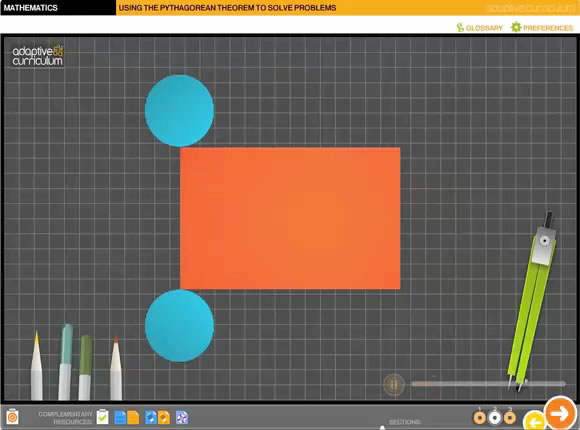Interactive Exercise
These Activity Objects provide learners with additional opportunities to develop conceptual understanding.
•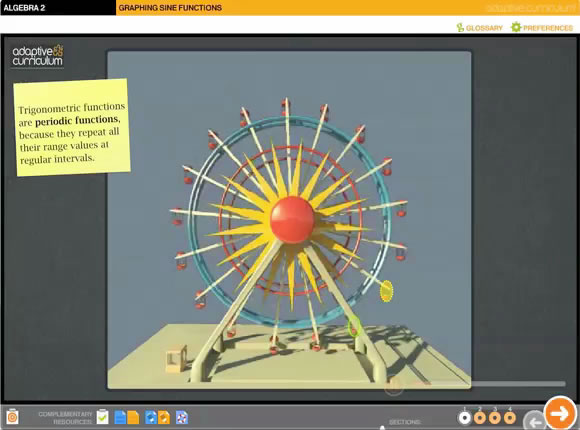Skills Application
Students directly apply mathematical skills to solve problems in mathematical and real-life contexts by using guided problem solving strategies and mathematical practices. Real-life problems are engaging and relevant to students, and mathematical problems are motivating with compelling visualizations and explanations.
•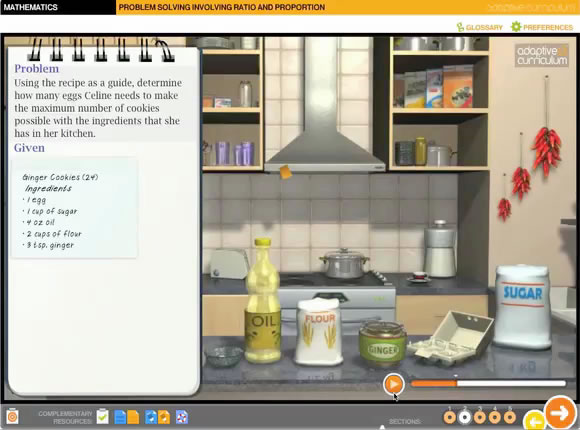Problem Solving
Students deepen their existing mathematical knowledge through active reasoning in guided inquiry and problem-solving environments. Students solve complicated or unstructured problems about the real world by using mathematical practices to form coherent strategies and conjectures. Students analyze whether solutions are reasonable, accurate, and correct.
•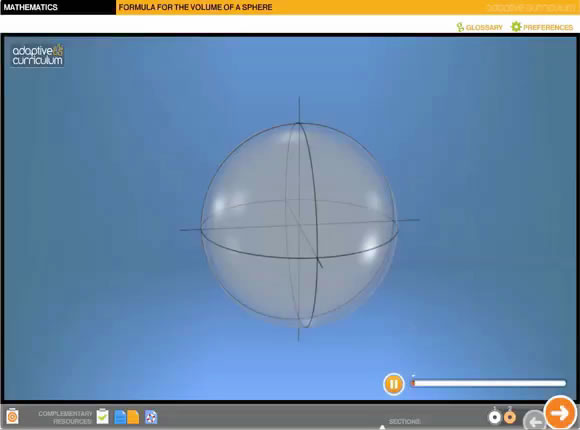Visual Proofs
Students synthesize and analyze mathematical concepts with compelling, multi-representational explanations and proofs. Students begin by making intuitive or contextual observations about mathematical facts, and then use active inquiry and mathematical reasoning to find solutions or proofs.

Browse our Catalog for hundreds of math Activity Objects to review their learner outcomes and performance objectives.

View the AC Math Scope for a list of Activity Objects organized by learning areas.

### AC Math

AC Math provides teachers with an instructional solution that promotes math mastery through dynamic, interactive learning. Unlike other programs developed for print, AC Math was developed as a digital solution to take full advantage of the online environment and cutting-edge instructional tools such as interactive whiteboards.

AC Math contains:

• Hundreds of Activity Objects (AOs) in grades 6-12
• An individual Teacher Guide for each AO
• Online end-of-activity assessments for each AO
• Activity sheets that can be printed for whole-class instruction
• My Adaptive Space for managing students and assignments
• Clear and intuitive assessment reports by student and assignment
• Alignments to Common Core and State standards
• Online or onsite product training

### Differentiation

AC Math can be presented on Interactive Whiteboards, used by small groups and assigned to individual students. Students can be assigned any math AO from grade 6 through high school (Algebra 2) depending on the learning needs, allowing teachers to truly differentiate instruction.

Check our AC Math catalog, our Math Activity Object Types or our AC Math Scope and Sequence.

### Teacher Support

Adaptive Curriculum's solutions are designed with the teacher in mind providing a variety of classroom teaching tools, including activity sheets, teacher guides, assessments and more!